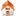# Spectral estimation on a sphere in geophysics and cosmology

## F. A. Dahlen and Frederik J Simons

### Geosciences Department Princeton University Princeton NJ 08544, USA

Geoph. J. Int., 2008, 174 (3), 774-807, doi:10.1111/j.1365-246X.2008.03854.x
 Reprint Editorial Related work Software Publications

#### Figures

1. Figure 01 Geometry of the problem
2. Figure 02 Power spectra of the single and the double polar boxcar caps for a varity of cap sizes
3. Figure 03 Power spectra of the single and the double polar boxcar cap with superimposed the asymptotic equivalent cap size wavelengths
4. Figure 04 Boxcar coupling kernels for various single and double cap sizes: perspective views
5. Figure 05 Boxcar coupling kernels for various single and double cap sizes [Log version]
6. Figure 06 Eigenvalue-weighted multitaper coupling kernels for various bandwidths: perspective views
7. Figure 07 Eigenvalue-weighted multitaper coupling kernels for various bandwidths [Log version]
8. Figure 08 Ratio of boxcar periodogram estimation variance over whole-sphere estimation variance
9. Figure 09 Ratio of maximum likelihood cut-sphere estimation variance over whole-sphere estimation variance
10. Figure 10 Ratio of eigenvalue-weighted multitaper estimation variance over whole-sphere estimation variance
11. Figure 11 Evolution of the eigenvalue-weighted multitaper variance ratio at large degrees
12. Figure 12 Cosmic Microwave Background radiation: spectra and uncertainties

Frederik Simons